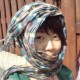# Triangulation!Triangulation.. Finally! Want it so bad for a semester. Luckily having a winter break to do it. Have a lot of rooms for improvement but still, one step further!

### *UPDATE*(in the middle of writing this post UGH)

New version. Normal speed!

In the middle of documentation, the ill performance, both low speed running and wrong color picking bothered me a lot, so I went through the whole codes again and making adjustment around. And then I found out what the problem is! It’s the random plotting of points for triangles! It not only slows down the process, but also causes the ill performance of choosing the right color for the triangle. OH YEAH SO HAPPY. It’s the similar “bitter yet sweet moment” I usually will have when coding… I think I’m ready for the new semester!

Old version. x3 speed! Slow and chaos.

Old Line version. Slow but it seems to have better outcome with the random plotting points methods!!

Image Gallery

Basic idea of code

1. capture image from webcam and save as PImage source
2. iterate through source, pick up every pixel, compare either a) colors or b) brightness difference(I found comparing by colors will be more accurate).
3. if the difference is bigger than certain threshold, plant a point in class Voronoi. Voronoi does all the calculation to transform points into triangles.
4. for each triangle gotten out from Voronoi, using getCentroid() to pickup the color to fill the triangle built up with beginShape(), endShape() and vertex().
5. wipe out Voronoi and build a new one every 10 frames to speed up the performance.

References

• from Robbie Tilton. ITP alumnus! This is where my base came from! Clear description made me not afraid of trying the code out! From him, I got the idea of using Cols and Rows to boost up the performance, and plotting points for triangles with a random deviation of 5 pixels to make it look less grid-like(BUT randomness is not good for picking color, and it also slows down the performance).
• from Jan Vantomme. Very well documentation! From him, I learned the difference between getCentroid() and getSites(), and also learned that, since getCentroid() and getSites() don’t return the voronoi regions and points in same order, to fill the right color for right position it has to be looped to iterate through, picking and filling up the color at the same time.

Inspiration & Further

Code, as below:

```//references:
//http://www.processing.org/tutorials/pixels/
//http://robbietilton.com/blog/?p=607
//http://vormplus.be/blog/article/voronoi-paintings-with-toxiclibs

import toxi.geom.*;
import toxi.geom.mesh2d.*;
import toxi.util.*;
import toxi.util.datatypes.*;
import toxi.processing.*;
import processing.video.*;

Capture video;
float size = 10000;
Voronoi voronoi;
PImage source;

int pointDensity = 70;
int cols, rows;
int x, y;
int numPixels;
float colorThreshold = 30;

void setup() {
size(640,480);
//size(source.width, source.height);
video = new Capture(this, width, height);
video.start();

source = createImage(640,480,RGB);
smooth();

cols = width/pointDensity;
rows = height/pointDensity;
numPixels = source.width * source.height;

voronoi = new Voronoi(size);

}

source.copy(video,0,0,640,480,0,0,640,480);
source.updatePixels();

for (x=1; x<source.width; x+=cols) {
for (y=1; y<source.height; y+=rows) {       int loc = x + y*source.width;       color pix = source.pixels[loc];              int leftLoc = (x-1) + (y-1)*source.width;       color leftPix = source.pixels[leftLoc];              // compare ver: color       float r1 = red(pix); float g1 = green(pix); float b1 = blue(pix);       float r2 = red(leftPix); float g2 = green(leftPix); float b2 = blue(leftPix);       float diff = dist(r1,g1,b1,r2,g2,b2);              // compare ver: brightness //      float diff = abs(brightness(pix)-brightness(leftPix));       if (diff > colorThreshold) {
//        voronoi.addPoint(new Vec2D(x+random(-2, 2), y+random(-2, 2)));
}
}
}
}

void drawTriangle(){

for (Triangle2D t : voronoi.getTriangles()) {
color pix;

// use Centroid's color
Vec2D locVec = t.computeCentroid();
int loc = (int)((locVec.x) + (locVec.y)*source.width);

pix = source.pixels[constrain(loc,0,numPixels-1)];
//    stroke(pix,100);
fill(pix);
//    noFill();
noStroke();

beginShape(TRIANGLES);
stroke(pix);
//    strokeWeight(.1);
vertex(t.a.x, t.a.y);
vertex(t.b.x, t.b.y);
vertex(t.c.x, t.c.y);
endShape();
}
}

void draw() {
background(255);
//image(video,0,0);

if(showImg) {
image(source, 0, 0);
} else {
drawTriangle();
}

fill(0);
rect(15,5,30,20);

// show frame rate
fill(255);
text(int(frameRate), 20, 20);

updatePixels();

if (frameCount%10 == 0)
voronoi = new Voronoi(size);

}

boolean showImg = false;
boolean saveImg = false;

void keyPressed() {
if(key == 'i')
showImg = !showImg;
}```

1.Photophiliac
•Laura